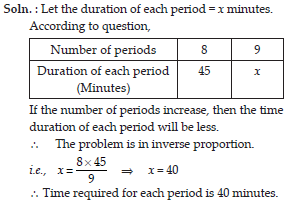Direct and Inverse Proportions (Mathematics) Class 8 - NCERT Questions
Q 1.

Following are the car parking charges near a railway station upto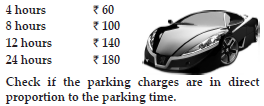SOLUTION: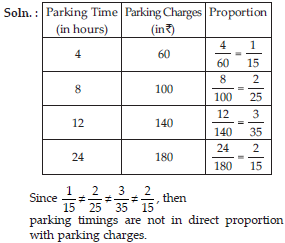Q 2.

A mixture of paints is prepared by mixing 1 part of red pigments with 8 parts of base. In the following table, find the parts of base that need to be added.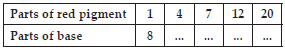SOLUTION: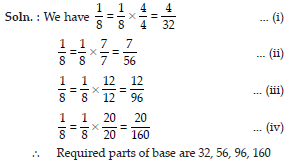Q 3.

In Question 2 above, if 1 part of a red pigment requires 75 mL of base, how much red pigment should we mix with 1800 mL of base?

SOLUTION: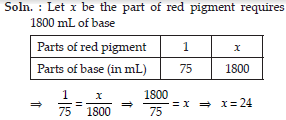Q 4.

A machine in a soft drink factory fills 840 bottles in six hours. How many bottles will it fill in five hours?

SOLUTION: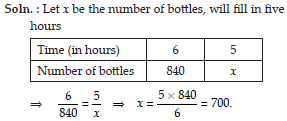Q 5.

A photograph of a bacteria enlarged 50,000 times attains a length of 5 cm as shown in diagram. What is the actual length of the bacteria? If the photograph is enlarged 20,000 times only, what would be its enlarged length?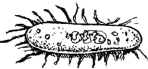SOLUTION: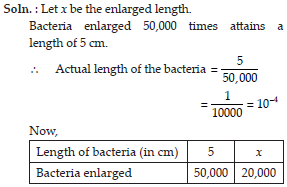Q 6.

In a model of a ship, the mast is 9 m high, while the mast of the actual ship is 12 m high. If the length of the ship is 28 m, how long is the model ship?SOLUTION: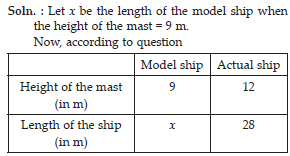Q 7.

Suppose 2 kg of sugar contains 9 × 106 crystals. How many sugar crystals are there in (i) 5 kg of sugar? (ii) 1.2 kg of sugar?

SOLUTION: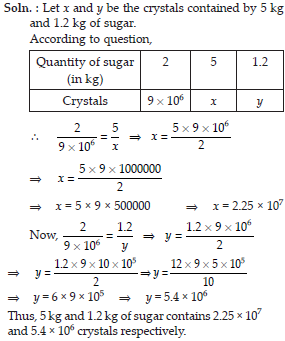Q 8.

Rashmi has a road map with a scale of 1 cm representing 18 km. She drives on a road of 72 km. What would be her distance covered in the map?

SOLUTION: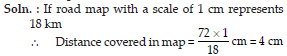Q 9.

A 5 m 60 cm high vertical pole casts a shadow 3 m 20 cm long. Find at the same time (i) the length of the shadow cast by another pole 10 m 50 cm high (ii) the height of a pole which casts a shadow 5 m long.

SOLUTION: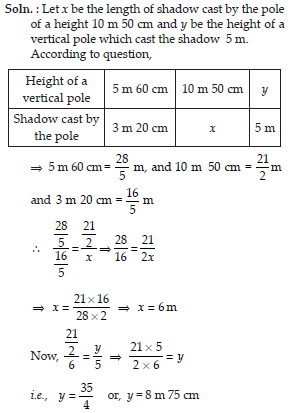Q 10.

A loaded truck travels 14 km in 25 minutes. If the speed remains the same, how far can it travel in 5 hours?

SOLUTION: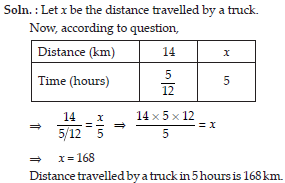Q 11.

Which of the following are in inverse proportion?
(i) The number of workers on a job and the time to complete the job.
(ii) The time taken for a journey and the distance travelled in a uniform speed.
(iii) Area of cultivated land and the crop harvested.
(iv) The time taken for a fixed journey and the speed of the vehicle.
(v) The population of a country and the area of land per person.

SOLUTION:

(i) If the number of workers on a job increase or decrease then the time to complete the job will decrease or increase.
∴ The given statement is in inverse proportion.
(ii) If the time taken for a journey increase or decrease then the distance travelled in uniform speed will also increase or decrease.
∴ The given statement is in direct proportion.
(iii) If the area of cultivated land increase or decrease then the crop harvested will also increase or decrease.
∴ The given statement is in a direct proportion.
(iv) If the time taken for a fixed journey increase, then the speed of the vehicle will decrease,but if the time taken for a fixed journey decrease, then the speed of the vehicle will increase.
∴ The given statement is in inverse proportion.
(v) If the population of a country increase then the area of land per person will decrease. But if the population of a country decrease then the area of land per person will increase.
∴ The given statement is in inverse proportion.

Q 12.

In a Television game show, the prize money of Rs.1,00,000 is to be divided equally amongst the winners. Complete the following table and find whether the prize money given to an individual winner is directly or inversely proportional to the number of winners?

SOLUTION: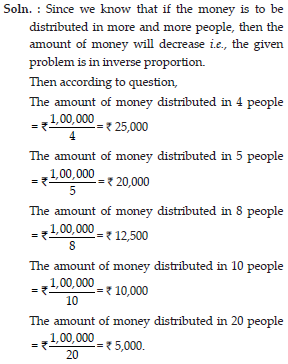Q 13.

Rehman is making a wheel using spokes. He wants to fix equal spokes in such a way that the angles between any pair of consecutive spokes are equal. Help him by completing the following table.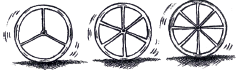SOLUTION: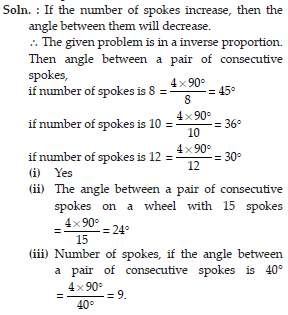Q 14.

If a box of sweets is divided among 24 children, they will get 5 sweets each. How many would each get, if the number of the children is reduced by 4?

SOLUTION: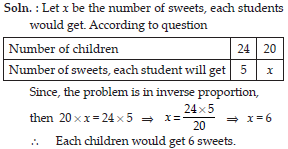Q 15.

A farmer has enough food to feed 20 animals in his cattle for 6 days. How long would the food last if there were 10 more animals in his cattle?

SOLUTION: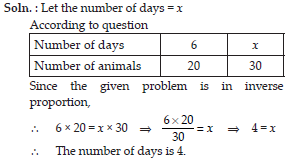Q 16.

A contractor estimates that 3 persons could rewire Jasminder’s house in 4 days. If, he uses 4 persons instead of three, how long should they take to complete the job?

SOLUTION: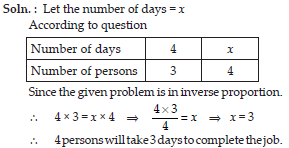Q 17.

A batch of bottles were packed in 25 boxes with 12 bottles in each box. If the same batch is packed using 20 bottles in each box, how many boxes would be filled?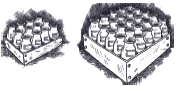SOLUTION: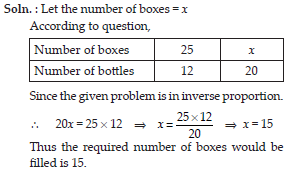Q 18.

A factory requires 42 machines to produce a given number of articles in 63 days. How many machines would be required to produce the same number of articles in 54 days?

SOLUTION: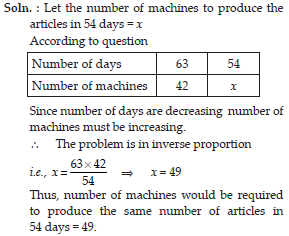Q 19.

A car takes 2 hours to reach a destination by travelling at the speed of 60 km/h. How long will it take when the car travels at the speed of 80 km/h?

SOLUTION: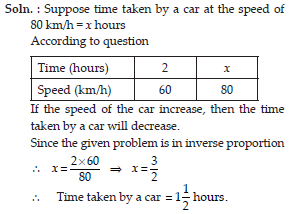Q 20.

Two persons could fit new windows in a house in 3 days.
(i) One of the persons fell ill before the work started. How long would the job take now?
(ii) How many persons would be needed to fit the window in one day?

SOLUTION: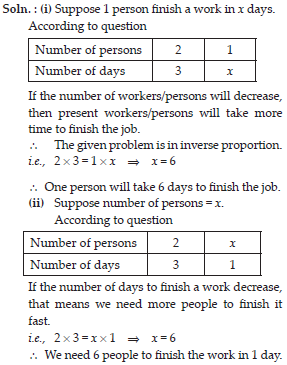Q 21.

A school has 8 periods a day each of 45 minutes duration. How long would each period be, if the school has 9 periods a day, assuming the number of school hours to be the same?

SOLUTION: